# Box plot in Plotly using graph_objects class

Plotly is a Python library which is used to design graphs, especially interactive graphs. It can plot various graphs and charts like histogram, barplot, boxplot, spreadplot and many more. It is mainly used in data analysis as well as financial analysis. plotly is an interactive visualization library.

## Box plot using graph objects class

If Plotly Express does not accommodate a good starting point, then it is also possible to use the more generic go.Box class from plotly.graph_objects. Box plot is a consistent way of distribution of data which are based on five main component shown below:

• Minimum : the lowest data point excluding any outliers.
• Maximum : the largest data point excluding any outliers.
• Median (Q2 / 50th Percentile) : the middle value of the dataset.
• First quartile (Q1 / 25th Percentile) : also known as the lower quartile qn(0.25), is the median of the lower half of the dataset.
• Third quartile (Q3 / 75th Percentile) : also known as the upper quartile qn(0.75), is the median of the upper half of the dataset.

Syntax: class plotly.graph_objects.Box(arg=None, alignmentgroup=None, boxmean=None, boxpoints=None, customdata=None, customdatasrc=None, dx=None, dy=None, fillcolor=None, hoverinfo=None, hoverinfosrc=None, hoverlabel=None, hoveron=None, hovertemplate=None, hovertemplatesrc=None, hovertext=None, hovertextsrc=None, ids=None, idssrc=None, jitter=None, legendgroup=None, line=None, lowerfence=None, lowerfencesrc=None, marker=None, mean=None, meansrc=None, median=None, mediansrc=None, meta=None, metasrc=None, name=None, notched=None, notchspan=None, notchspansrc=None, notchwidth=None, offsetgroup=None, opacity=None, orientation=None, pointpos=None, q1=None, q1src=None, q3=None, q3src=None, quartilemethod=None, sd=None, sdsrc=None, selected=None, selectedpoints=None, showlegend=None, stream=None, text=None, textsrc=None, uid=None, uirevision=None, unselected=None, upperfence=None, upperfencesrc=None, visible=None, whiskerwidth=None, width=None, x=None, x0=None, xaxis=None, xcalendar=None, xsrc=None, y=None, y0=None, yaxis=None, ycalendar=None, ysrc=None, **kwargs)

Parameters:
x: Sets the x sample data or coordinates. See overview for more info.
y: Sets the y sample data or coordinates. See overview for more info.
hoverinfo: Determines which trace information appear on hover.
marker: Instance or dict with compatible properties.
mean: Sets the mean values.
median: Sets the median values.

Example:

## Python3

 `import` `plotly.graph_objects as px ` `import` `numpy as np ` ` `  ` `  `# creating random data through randomint  ` `# function of numpy.random  ` `np.random.seed(``42``) ` ` `  `random_y1``=` `np.random.randint(``1``,``101``,``100``)  ` `random_y2``=` `np.random.randint(``1``,``101``,``100``) ` ` `  `x ``=` `[``'A'``, ``'B'``, ``'C'``, ``'D'``] ` ` `  `plot ``=` `px.Figure() ` ` `  `plot.add_trace(px.Box(y``=``random_y1)) ` `plot.add_trace(px.Box(y``=``random_y2)) ` ` `  `plot.show()`

Output: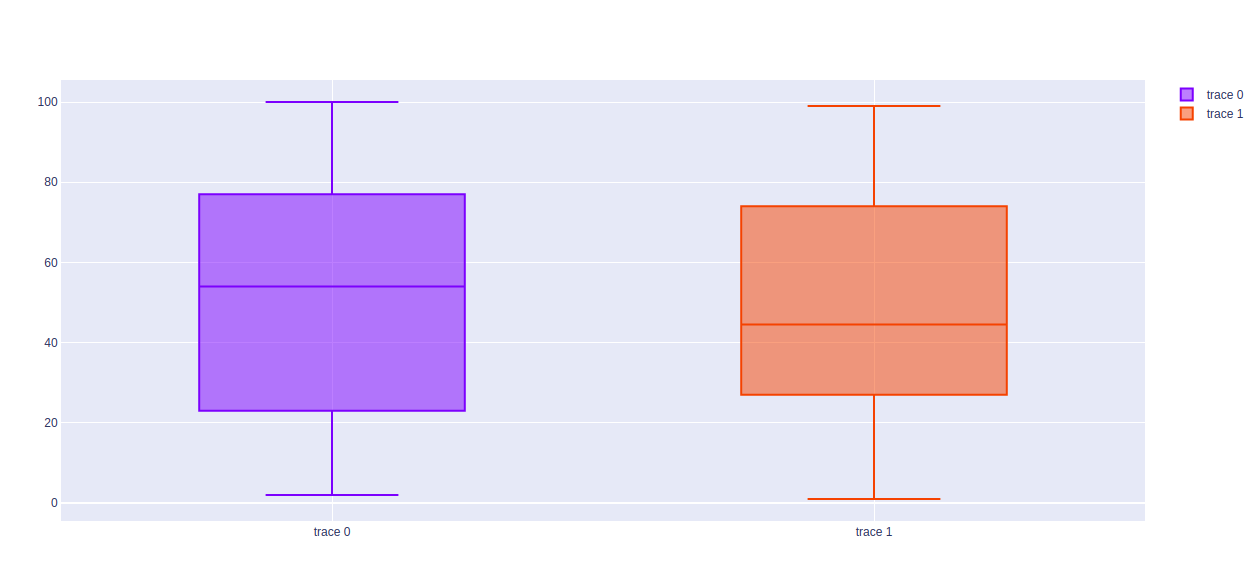## Horizontal Box Plot

The horizontal box plot is a box plot in which the x-variable and y-values are shown horizontally in the graph. It can be created by passing the x argument of the box plot.

Example: Use the x argument for horizontal graphs

## Python3

 `import` `plotly.graph_objects as px ` `import` `numpy as np ` ` `  ` `  `# creating random data through randomint  ` `# function of numpy.random  ` `np.random.seed(``42``) ` ` `  `random_x1``=` `np.random.randint(``1``,``101``,``100``)  ` `random_x2``=` `np.random.randint(``1``,``101``,``100``) ` ` `  `x ``=` `[``'A'``, ``'B'``, ``'C'``, ``'D'``] ` ` `  `plot ``=` `px.Figure() ` ` `  `plot.add_trace(px.Box(x``=``random_x1)) ` `plot.add_trace(px.Box(x``=``random_x2)) ` ` `  `plot.show()`

Output: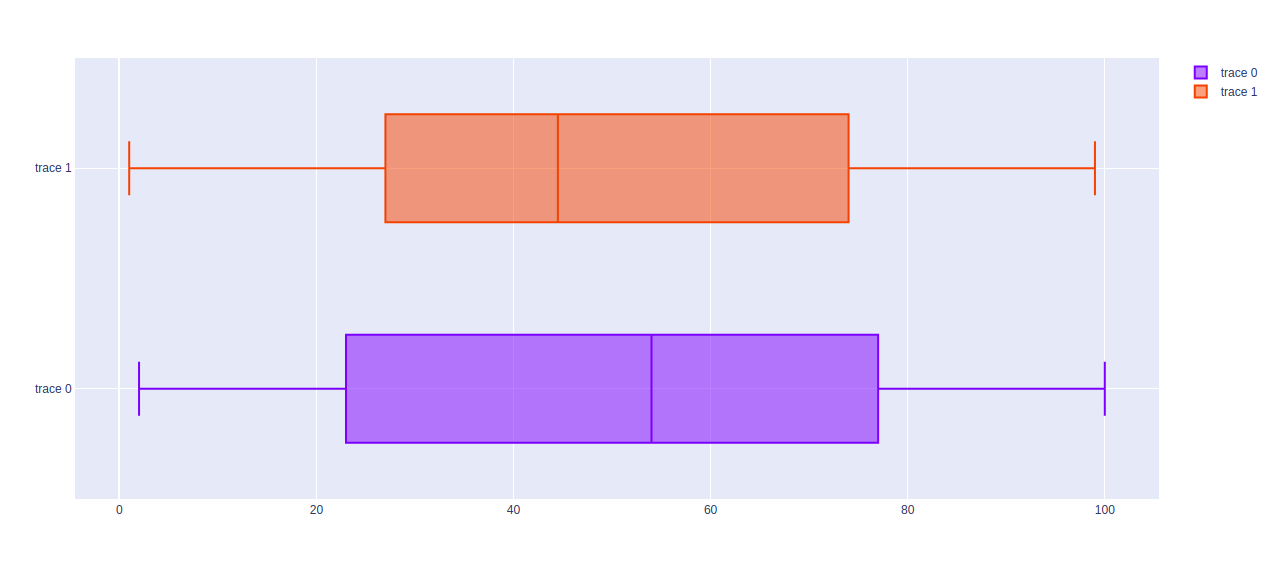## Finding Mean and Standar Deviation

The mean and standard deviation of the data plotted by boxplot can be found using the boxmean parameter. It can take two values –

• True for mean
• sd for standard deviation.

Example:

## Python3

 `import` `plotly.graph_objects as px ` `import` `numpy as np ` ` `  ` `  `# creating random data through randomint  ` `# function of numpy.random  ` `np.random.seed(``42``) ` ` `  `random_x1``=` `np.random.randint(``1``,``101``,``100``)  ` `random_x2``=` `np.random.randint(``1``,``101``,``100``) ` ` `  `x ``=` `[``'A'``, ``'B'``, ``'C'``, ``'D'``] ` ` `  `plot ``=` `px.Figure() ` ` `  `plot.add_trace(px.Box(x``=``random_x1, marker_color ``=` `'indianred'``, boxmean``=``True``)) ` `plot.add_trace(px.Box(x``=``random_x2, marker_color``=``'royalblue'``, boxmean``=``'sd'``)) ` ` `  `plot.show()`

Output: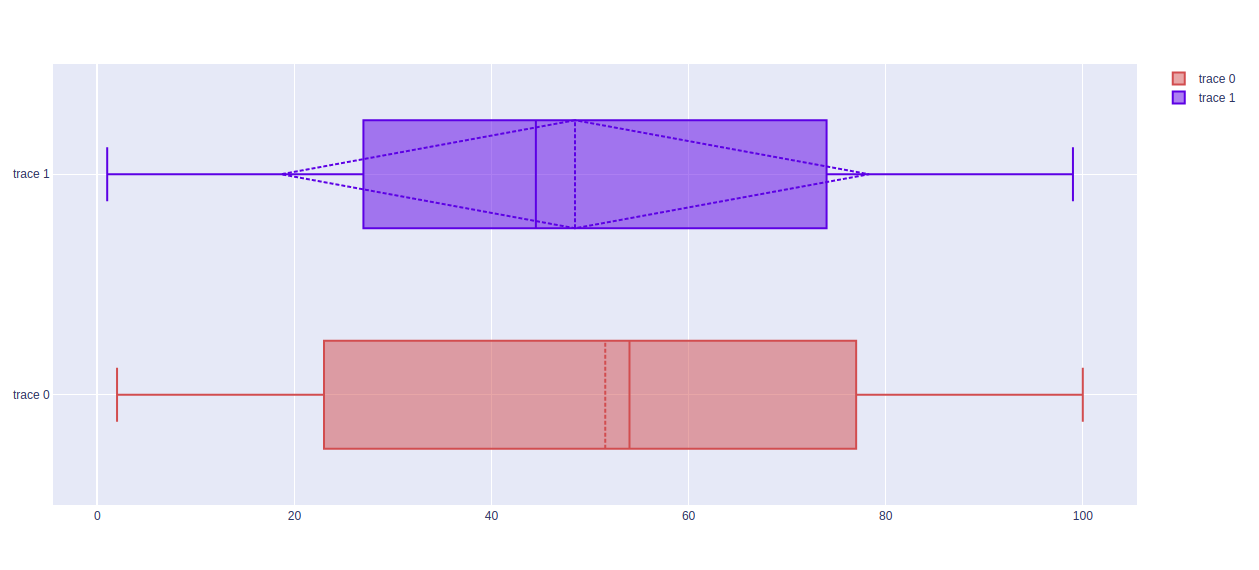## Changing Algorithms for Quartiles

The algorithm to choose quartiles can also be selected in plotly. It is computed by using linear algorithm by default. However, it provides two more algorithms for doing the same i.e. inclusive and exclusive. It can be done by passing the quartilemethod argument.

Example 1:

## Python3

 `import` `plotly.graph_objects as px ` `import` `numpy as np ` ` `  ` `  `# creating random data through randomint  ` `# function of numpy.random  ` `np.random.seed(``42``) ` ` `  `random_y``=` `np.random.randint(``1``,``101``,``100``) ` ` `  `x ``=` `[``'A'``, ``'B'``, ``'C'``, ``'D'``] ` ` `  `plot ``=` `px.Figure() ` ` `  `plot.add_trace(px.Box(y``=``random_y, quartilemethod``=``"linear"``, name``=``"linear"``)) ` `plot.add_trace(px.Box(y``=``random_y, quartilemethod``=``"inclusive"``, name``=``"inclusive"``)) ` `plot.add_trace(px.Box(y``=``random_y, quartilemethod``=``"exclusive"``, name``=``"exclusive"``)) ` ` `  `plot.show()`

Output: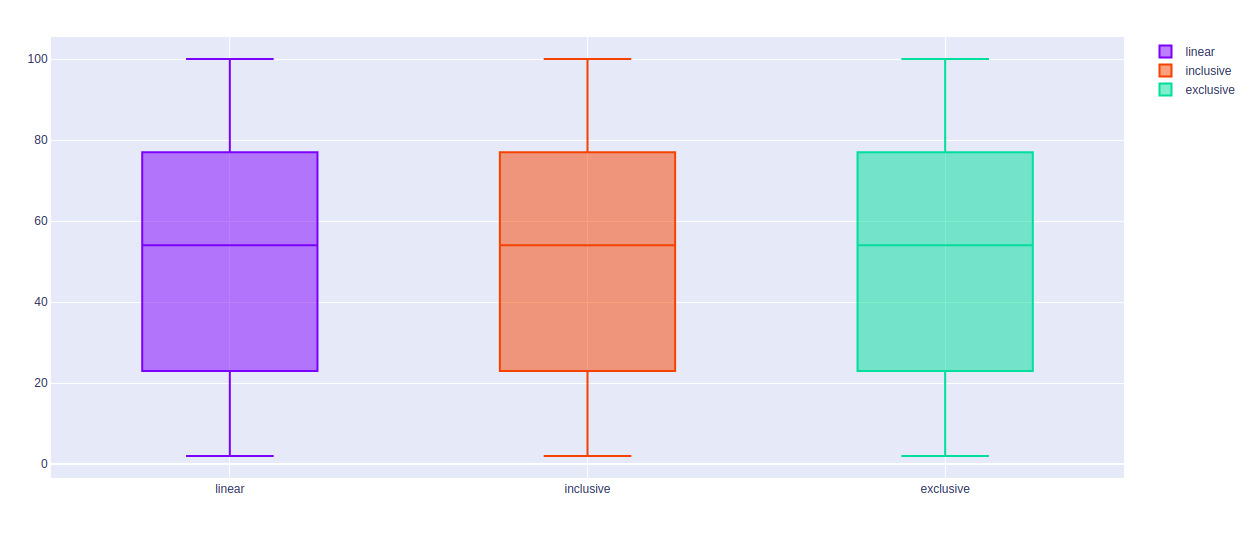## Showing The Underlying Data

Underlying data can be shows using the boxpoints arguments. The value of this argument can be of three types –

• all for all points
• outliers for outliers only
• false for none of the above

Example:

## Python3

 `import` `plotly.graph_objects as px ` `import` `numpy as np ` ` `  ` `  `# creating random data through randomint  ` `# function of numpy.random  ` `np.random.seed(``42``) ` ` `  `random_y1``=` `np.random.randint(``1``,``101``,``100``)  ` `random_y2``=` `np.random.randint(``1``,``101``,``100``) ` ` `  `x ``=` `[``'A'``, ``'B'``, ``'C'``, ``'D'``] ` ` `  `plot ``=` `px.Figure() ` ` `  `plot.add_trace(px.Box(y``=``random_y1, boxpoints``=``"all"``)) ` `plot.add_trace(px.Box(y``=``random_y2, boxpoints``=``"outliers"``)) ` ` `  ` `  `plot.show()`

Output: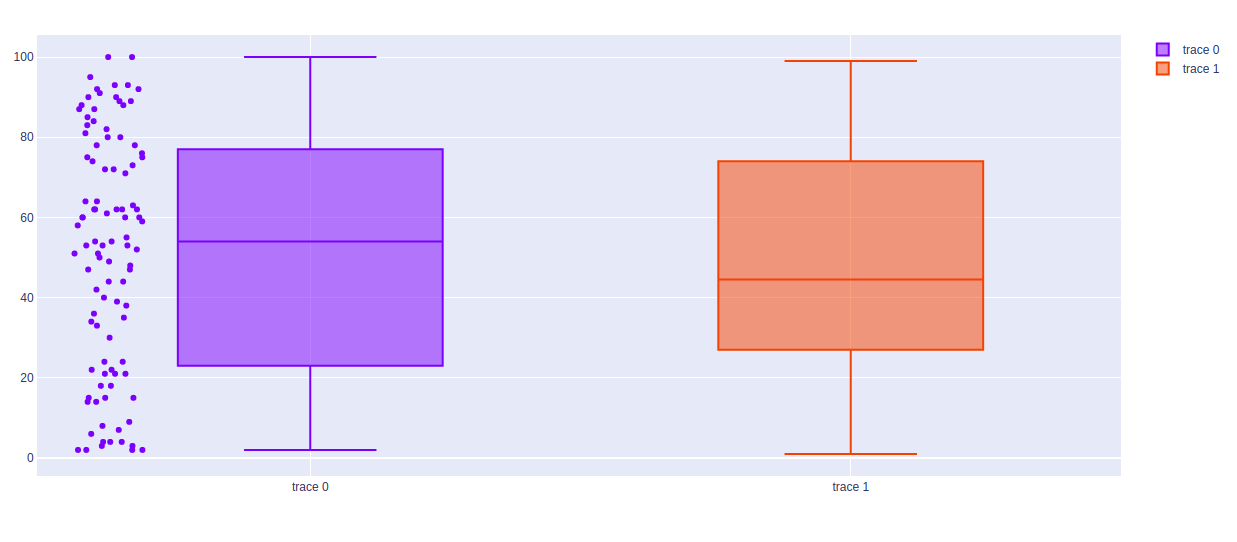My Personal Notes arrow_drop_upIf you like GeeksforGeeks and would like to contribute, you can also write an article using contribute.geeksforgeeks.org or mail your article to contribute@geeksforgeeks.org. See your article appearing on the GeeksforGeeks main page and help other Geeks.

Please Improve this article if you find anything incorrect by clicking on the "Improve Article" button below.

Article Tags :

Be the First to upvote.

Please write to us at contribute@geeksforgeeks.org to report any issue with the above content.